# Upper-and-lower-functions method

A method for demonstrating the existence of solutions of boundary value problems for differential equations. The idea of this method applied to ordinary differential equations was discussed in the work of G. Peano (1880). For the case of the Dirichlet problem and for the case of the Laplace equation the idea occurs in H. Poincaré's balayage method. O. Perron  was the first to give a full exposition of the method of upper and lower functions for this last case.

Let the Dirichlet problem be posed in a regionof the space,, for a linear, homogeneous, elliptic second-order equation with continuous coefficients,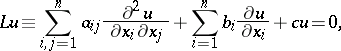(1)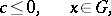with the boundary condition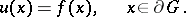(2)

In the method of upper and lower functions one introduces a generalization of superharmonic functions (respectively, of subharmonic functions), under the assumption that the problems (1) and (2) are locally solvable. A functionwhich is continuous inis said to be a generalized superharmonic function (respectively, a generalized subharmonic function) inif for any sufficiently small ball,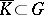, the inequality(respectively,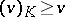) is true. Here,is a continuous function inwhich is equal tooutsideand on its boundary and which satisfies equation (1) inside. For a continuous functionon the boundary, a generalized superharmonic (respectively, a subharmonic) functionis said to be upper (respectively, lower) if for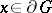the inequality(respectively,) is true.

The classes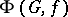and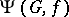of all upper and lower functions, respectively, are non-empty, and if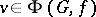and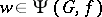, then. A generalized solution of the Dirichlet problem is defined as the smallest envelope of the classor as the largest envelope of the class:(3)If the boundarypermits a barrier at each one of its points, then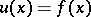everywhere on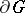, i.e.is a classical solution of the Dirichlet problem. In the general case the behaviour of the generalized solution (3) of the elliptic equation (1) at boundary points fully parallels the behaviour of the generalized solution of the Laplace problem (cf. Perron method).

The method of upper and lower functions is also employed in the study of the first boundary value problem for linear homogeneous parabolic second-order equations of the form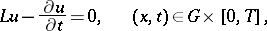with the initial condition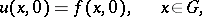and boundary condition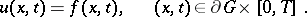In this case superparabolic (subparabolic) functions, with properties analogous to those of generalized superharmonic (subharmonic) functions, are introduced .